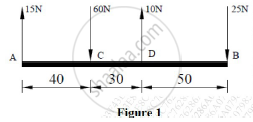# Find the Resultant of the Parallel Force System Shown in Figure 1 and Locate the Same with Respect to Point C. ( - Engineering Mechanics

Find the resultant of the parallel force system shown in Figure 1 and locate the same with respect to point C.#### Solution

ΣFx = 0→ +ve
ΣFy = 15 - 60 + 10 -25 ↑ +ve
= -60 N
R =sqrt(Fx^2 + Fy^2)
= sqrt((−60)
R = 60 N
Ɵ = tan^-1((∑Fy)/(∑Fx))
tan^-1 (-60/0)

=-90°

By Varignon’s theorem,
ΣMcF = R x d +ve

d is the distance of the resultant from point C and assume R to be on the right of point C
(15x40) – (10x30) + (25x80) = 60xd
d = 38.333 m

The resultant force is 60 N downwards and is located 38.333 m away to the right of point C.

Concept: Resultant of parallel forces
Is there an error in this question or solution?